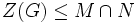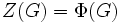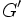# Classification of finite non-abelian groups in which every proper subgroup is abelian

## Related facts

Here are some easier-to-prove facts about these finite non-abelian groups. The easier-to-prove facts are not used directly in our proof but the proof ideas for those facts are extended here. Note that these easier facts do not require a knowledge of Frobenius groups:

## Facts used

1. Center is normal
2. Fourth isomorphism theorem
3. Abelianness is quotient-closed
4. Maximal implies normal or self-normalizing
5. The theorem about the equivalent definitions of Frobenius group.
6. Cyclic over central implies Abelian

## Proof

### First step: establishing properties of the inner automorphism group

We shall prove that the inner automorphism group, i.e., the quotient of the group by its center, must be either an Abelian group of a Frobenius group. If it is an Abelian group, the problem reduces to classifying all groups of nilpotence class two in which every proper subgroup is Abelian. We can also tackle the case of the Frobenius group.

Given: A finite non-Abelian group$G$ in which every proper subgroup is Abelian.$Z(G)$ is the center of$G$, and$G/Z(G)$ is non-Abelian.

To prove:$G/Z(G)$ is a Frobenius group.

Proof:

1. If$M,N$ are two distinct maximal subgroups of$G$ containing$Z(G)$, then$M \cap N = Z(G)$: By assumption, both$M$ and$N$ are Abelian, so$M \cap N$ is centralized by both$M$ and$N$. Hence,$M \cap N$ is centralized by$\langle M, N \rangle = G$. Thus,$M \cap N \le Z(G)$. On the other hand, we have$Z(G) \le M, Z(G) \le N$ by assumption, so$Z(G) \le M \cap N$. Thus,$M \cap N = Z(G)$.
2. In the quotient group$G/Z(G)$, any two distinct maximal subgroups intersect trivially: This follows from step (1) and fact (2) (the fourth isomorphism theorem).
3. There exists a maximal subgroup that is not normal: If every maximal subgroup is normal, we have two possibilities: either there is a unique maximal subgroup, or there are two distinct maximal normal subgroups.
• If there is a unique maximal subgroup, then any element outside the maximal subgroup generates the whole group, and hence$G$ itself is cyclic, and in particular,$G/Z(G)$ is non-Abelian.
• If there are two distinct maximal normal subgroups, we get two normal subgroups of$G/Z(G)$ that intersect trivially (step (1)) and generate the whole group; moreover, by fact (3), they are both images of Abelian subgroups and hence Abelian. Thus,$G/Z(G)$ is a direct product of two Abelian subgroups, and is Abelian, a contradiction.
4. Suppose$A$ is a maximal subgroup of$G/Z(G)$ that is not normal. Then$A$ is a Frobenius complement in$G/Z(G)$: Since$A$ is maximal and not normal,$A$ is self-normalizing (fact (4)). In particular,$A$ is self-centralizing, and thus,$A$ does not equal its conjugate by any element outside$A$. By step (1), we conclude that$A$ intersects its conjugate by any other element trivially, fulfilling the conditions for a Frobenius complement.

### Classification of the nilpotent groups

We now consider the case of a finite non-Abelian group$G$ of nilpotence class two in which every proper subgroup is Abelian.

1.$G$ is a group of prime power order, where we denote the prime by$p$: Any finite nilpotent group is a direct product of its Sylow subgroups. If the order of$G$ has more than one prime factor, then all its Sylow subgroups are proper subgroups, and by assumption, each of the Sylow subgroups is Abelian. Thus,$G$ is a direct product of Abelian groups, and is Abelian, contradicting the assumption.
2. If$K \le G$ is such that$G/K$ is elementary Abelian of order$p^2$, then$K \le Z(G)$: Since$G/K$ is elementary Abelian,$K$ is the intersection of two maximal subgroups of$G$, both of which are Abelian by assumption. The intersection of these maximal subgroups is centralized by

both of them, and hence,$K$ is centralized by$G$.

1. The center of$G$ has index at least$p^2$: Since$G$ is non-Abelian, the center has index at least$p$. If the index is$p$, fact (6) yields that since the group is cyclic over its center, it is Abelian, again a contradiction. Thus, the center has index at least$p^2$.
2.$Z(G) = \Phi(G)$ has index$p^2$: If$\Phi(G)$ has index$p$, then$G$ has a unique maximal subgroup, hence is cyclic (on any element outside the maximal subgroup) and therefore Abelian by assumption. Thus,$\Phi(G)$ has index at least$p^2$. If the index of$\Phi(G)$ is greater than$p^2$, then there are multiple subgroups of$G$ with quotient elementary Abelian of order$p^2$, and all of these are in the center of$G$ by step (2). This forces the center to have index less than$p^2$, contradicting step (3). Thus, the index of$\Phi(G)$ is$p^2$. Again by step (2), this gives$\Phi(G) \le Z(G)$, and step (3) yields$\Phi(G) = Z(G)$.
3. The commutator subgroup$G'$ is cyclic of prime order: The commutator subgroup is the image of$G/Z(G) \otimes G/Z(G)$ under the commutator map, which is an alternating bilinear map. Since the vector space in question is two-dimensional, we see that$G'$ has dimension at most one (another way of saying this is that it suffices to know the commutator of any two distinct basis vectors of$G/Z(G)$). Since$G$ is non-Abelian, we obtain that$G'$ is one-dimensional as a vector space over the field of$p$ elements, yielding that$G'$ is cyclic of order$p$.

We now have enough background information to guess at a classification, that we will then prove.